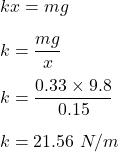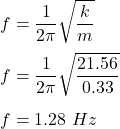## A 50-cm-long spring is suspended from the ceiling. A 330 g mass is connected to the end and held at rest with the spring unstretched. The ma

Question

A 50-cm-long spring is suspended from the ceiling. A 330 g mass is connected to the end and held at rest with the spring unstretched. The mass is released and falls, stretching the spring by 30 cm before coming to rest at its lowest point. It then continues to oscillate vertically.

A. What is the spring constant?

B. What is the amplitude of the oscillation?

C. What is the frequency of the oscillation?

in progress 0
5 months 2021-08-05T01:12:45+00:00 1 Answers 43 views 0

## Answers ( )

1. Explanation:

Given that,

Length of the spring, l = 50 cm = 0.5 m

Mass connected to the end, m = 330 g = 0.33 kg

The mass is released and falls, stretching the spring by 30 cm before coming to rest at its lowest point. On applying Newton’s second law, 10 cm below the release point, x = 15 cm

(a) When the mass is connected, the force of gravity is balanced by the force in spring.(b) The amplitude of the oscillation will be 15 cm as it is half of the total distance travelled.

(c) The frequency of the oscillation is given by :Hence, this is the required solution.